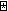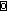﻿ Power Operator Extension

An Extension to the Power Operator

E.E. McDonnell

I.P. Sharp Associates
425 Sherman Avenue
Palo Alto, CA 94306-1827

The dyadic power operator, producing a monadic derived function, has been discussed in APL circles for many years. The earliest published discussion of which I am aware appeared in “General Arrays, Operators, and Functions”, by Ziad Ghandour and Jorge Mezei, which appeared in IBM Journal of Research and Development, 17, 4, July 1973. In this paper the operator was denoted by. The operator was later discussed in the IBM Technical Report RC 7091, Operators and Functions, by K.E. Iverson, April 1978, pp 2-3. The NARS system developed by Bob Smith at STSC in 1981 included the power operator, denoted, as Iverson’s paper had suggested, by the symbol . The STSC Reference Manual Nested Array System, by Carl M. Cheney, 1981, pp 44-48, gives several examples of its use. Cheney gives the example of using the power operator with the function

`   Fib: ⍵,+/¯2↑⍵`

as follows:

`   (Fib ⍣ 11) 1 1`

yields the thirteen Fibonnaci numbers

`1 1 2 3 5 8 13 21 34 55 89 144 233`

In this recently published “A Dictionary of APL”, APL Quote Quad 18 1, September 1987, Iverson renews the discussion of this operator, now denoted by the dot (because of the ambiguities that arise from the fact that the dot is used not only as an operator symbol but in forming numbers and names, a space may be required to separate the left or right argument from the dot). He gives the definition of f . n , where f is a monadic function and n is an integer, as follows:

 The function f is applied n times. For example: ```⍟. 2 ←→ ⍟⍟ ⍵ -⌿. 4 ←→ -⌿-⌿-⌿-⌿ ⍵ 1¨○. 3 ←→ 1○1○1○ ⍵```

In all the prior discussions of this operator, it has been the case that its derived function was monadic. The purpose of this note is to suggest a use for a dyadic derived function.

In IEEE Software 3, 1, January, 1986, an article “FAC: A Functional APL Language”, by Hai-Chen Tu and Alan J. Perlis, appeared. In it, the authors, in the course of a discussion on infinitely large arrays in APL, introduced the iter operator, which they denoted. They note that it derives to an ambivalent function. They define (F) A to mean:

`A, (F A), (F (F A)), (F (F (F A))), ...`

so that the nth element of the result would be equivalent to Iverson’s F.n A . They give the definition of W: FA recursively as defining an infinite vector W such that W = A , and in general, W[i+1] = (F W[i])

More importantly, for the purposes of this note, they also define a dyadic derived case, A (F) B , to mean

`A, B, (A F B), (B F (A F B)), ((A F B) F (B F (A F B))), ...`

Recursively, they define W: A (F) B to mean an infinite vector W such that W is A , W is B , and in general, W[i+2] is W[i] F W[i+1] . They give the example

```   1 +1
1 1 2 3 5 8 ...
```

the infinite Fibonacci sequence.

We can take this dyadic derive case of Tu and Perlis from the infinite realm to currently finite APL, by adding the dyad ⍺ f . n ⍵ to Iverson’s monad case f . n ⍵ . The definition of ⍺ f . n ⍵ is:

```   n       ⍺ f . n ⍵

0       ⍺
1       ⍵
2       ⍺ f ⍵
3       ⍵ f ⍺ f ⍵
...
n       (⍺ f .(n-2)⍵) f ⍺ f .(n-1)⍵
```

For example, the first fifteen Fibonacci numbers are given by 0 +.(⍳15) 1 . The 19th power of the golden mean number phi=0.6180339887... is given by 1 -. 19 phi . For the function Fact: ⍵×1+⍵÷⍺ the expression 1 Fact . n 1 gives the value !n , for any array of nonnegative integers n .

E.E. McDonnell
I.P. Sharp Associates
Palo Alto, California

I should like to give thanks to Cory Skutt, of IBM Research in Yorktown Heights, NY, for calling the Tu-Perlis article to my attention, to K.E. Iverson for suggesting the possibility of using nonscalar arrays n as right arguments, and to Bob Smith for his careful reading of an early draft of this note, which led to several useful comments and corrections.

First appeared in APL Quote-Quad, Volume 18, Number 4, 1988-06, in an article dated 1988-05-04.

 created: 2009-09-29 20:55 updated: 2013-09-23 21:40